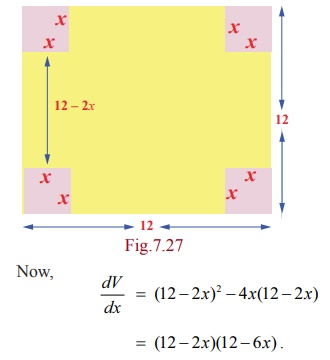Home | | Maths 12th Std | Applications in Optimization

# Applications in Optimization

Optimization is a process of finding an extreme value (either maximum or minimum) under certain conditions.

Applications in Optimization

Optimization is a process of finding an extreme value (either maximum or minimum) under certain conditions.

A procedure for solving for an extremum or optimization problems.

Step 1 : Draw an appropriate figure and label the quantities relevant to the problem.

Step 2 : Find a experssion for the quantity to be maximized or minimized.

Step 3 : Using the given conditions of the problem, the quantity to be extremized .

Step 4 : Determine the interval of possible values for this variable from the conditions given in the problem.

Step 5 : Using the techniques of extremum (absolute extrimum, first derivative test or second derivative test) obtain the maximum or minimum.

Example 7.62

We have a 12 unit square piece of thin material and want to make an open box by cutting small squares from the corners of our material and folding the sides up. The question is, which cut produces the box of maximum volume?

Solution

Let x = length of the cut on each side of the little squares.

V = the volume of the folded box.

The length of the base after two cuts along each edge of size x is 12 ŌłÆ 2x . The depth of the box after folding is x , so the volume is V = x ├Ś (12 ŌłÆ 2x)2 . Note that, when x = 0 or 6 , the volume is zero and hence there cannot be a box. Therefore the problem is to maximize, V = x ├Ś (12 ŌłÆ 2x )2 , x Ōłł(0, 6).Now, dV / dx = (12 ŌłÆ 2x)2 ŌłÆ 4x(12 ŌłÆ 2x)

= (12 ŌłÆ 2x )(12 ŌłÆ 6x).

dV / dx = 0 gives the stationary numbers x = 2, 6 . Since  6Ōłē(0,6) the only stationary number is at x = 2 Ōłł(0, 6) . Further, dV  / dx  changes its sign from postive to negative when passing through x = 2 . Therefore at x = 2 the volume V is local maximum. The local maximum volume value is V = 128 units. Hence the maximum cut can only be 2 units.

### Example 7.63

Find the points on the unit circle x2 + y2 = 1 nearest and farthest from (1,1) .

Solution

The distance from the point (1,1) to any point ( x , y) is d = ŌłÜ{(x ŌłÆ1)2 + ( y ŌłÆ1)2} . Instead of extremising d, for convenience we extremise D = d2 = (x ŌłÆ 1)2 + (y ŌłÆ1)2, subject to the condition x2 + y2 =1. Now, dD/dx = 2(x ŌłÆ 1) + 2( y ŌłÆ1) ├Ś dy/dx , where the dy/dx will be computed by differentiating x2 + y2 = 1 with respect to x . Therefore, we get 2x + 2y dy/dx = 0 which gives us dy/dx = ŌłÆ x/y .Since ( x , y) lie on the circle x2 + y2 =1, we get 2x2 =1 which gives x = ┬▒ 1/ŌłÜ2 . Hence the points at which the extremum distance occur are, (1/ŌłÜ2, 1/ŌłÜ2), (-1/ŌłÜ2, -1/ŌłÜ2).

To find the extrema, we apply second derivative test. So,This implies the nearest and farthest points are (1/ŌłÜ2, 1/ŌłÜ2) and (-1/ŌłÜ2, -1/ŌłÜ2) respectively.

Therefore, the nearest and the farthest distances are respectively ŌłÜ2 - 1 and ŌłÜ2 + 1.

### Example 7.64

A steel plant is capable of producing x tonnes per day of a low-grade steel and y tonnes per day of a high-grade steel, where y = 40ŌłÆ5x  / 10-x or []. If the fixed market price of low-grade steel is half that of high-grade steel, then what should be optimal productions in low-grade steel and high-grade steel in order to have maximum receipts.

### Solution

Let the price of low-grade steel be Ōé╣p per tonne. Then the price of high-grade steel is Ōé╣2p per tonne.

The total receipt per day is given by R = px + 2py = px + 2p[40ŌłÆ5x  / 10-x]. Hence the problem is to maximise R . Now, simplifying and differentiating R with respect to x , we getand hence R will be maximum. If x = 10-2ŌłÜ5 then y = 5 ŌłÆ ŌłÜ5 . Therefore the steel plant must produce low-grade and high-grade steels respectively in tonnes per day are

10-2ŌłÜ5 and 5 ŌłÆ ŌłÜ5.

### Example 7.65

Prove that among all the rectangles of the given area square has the least perimeter.

Solution

Let x , y be the sides of the rectangle. Hence the area of the rectangle is xy = k (given). The perimeter of the rectangle P is 2(x + y) . So the problem is to minimize 2(x + y) suject to the condition xy = k . Let P (x) = 2 (x + k/x).P ŌĆ▓(x) = 0 ŌćÆ x = ┬▒ŌłÜk.

As x, y are sides of the rectangle, x = ŌłÜk is a critical number.

Now, P ŌĆ▓ŌĆ▓ (x) = 4k/ x3 and P ŌĆ▓ŌĆ▓ ( ŌłÜk ) > 0 ŌćÆ P ( x) has its minimum value at x = ŌłÜk .

Substituting x = ŌłÜk in xy = k we get y = ŌłÜk . Therefore the minimum perimeter rectangle of a given area is a square.

Tags : Applications of Differential Calculus | Mathematics , 12th Maths : UNIT 7 : Applications of Differential Calculus
Study Material, Lecturing Notes, Assignment, Reference, Wiki description explanation, brief detail
12th Maths : UNIT 7 : Applications of Differential Calculus : Applications in Optimization | Applications of Differential Calculus | Mathematics